# SSC CGL 2013 Tier 1 19 May Morning

Instructions

For the following questions answer them individually

Question 191

In the following diagram, the triangle represents Mothers, circle represents Teachers and the rectangle stands for Women. Which letter (out of A, B, C and D) represents women who are mothers as also teachers ?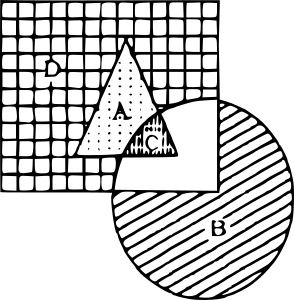Question 192

Which of the answer figures is not made up of only the components of the key figure.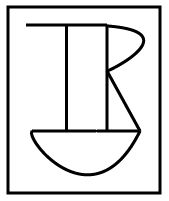Question 193

Which one of the following diagrams represents the correct relationship among Poison, Bio-products and Food ?

Question 194

In the given figure the triangle represents people who visited Mysore; the circle represents people who visited Ooty, the square represents people who visited Munnar. The portion which represents people who visited both Mysore and Ooty is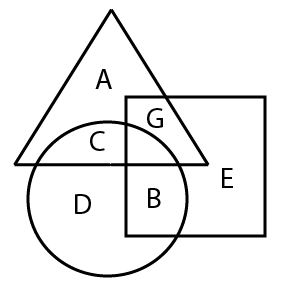Instructions

In the following questions, which answer figure will complete the pattern in the question figure.

Question 195

Find out which answer figures will exactly make up the question figure ?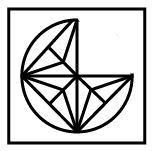Question 196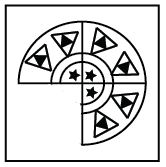Question 197

From the answer figures, find out the figure which is the exact mirror image of the question figure, when the mirror is placed on the line ‘MN’.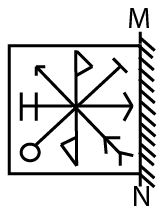Question 198

A word is represented by only one set of numbers as given in any one of the alternatives. The sets of numbers given in the alternatives are represented by two classes of alphabets as in the matrix given below. The columns and rows of Matrix are numbered from 0 to 6. A letter from the matrix can be represented first by its row and next by its column, e,g., ‘A’ can be represented by 42, 62, etc. and ‘P’ can be represented by 15, 43, etc. Similarly, you have to identify the set for the word ‘SNOW’.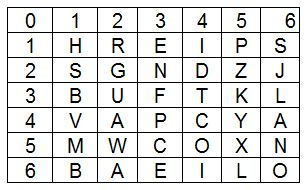Question 199

From the given answer figures, select the one in which the question figure is hidden/embedded.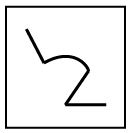Question 200

A piece of paper is folded and punched as shown below in the question figures. From the given answer figures, indicate how. it will appear when opened.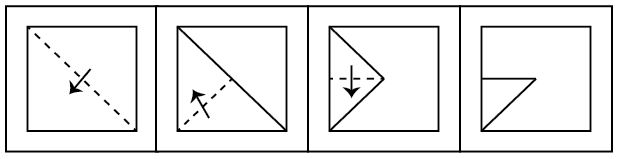OR